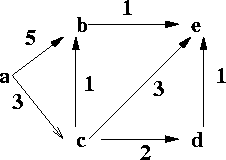CSE 5311 Fall 1999

Quiz #9

1.
(14 points). Compute the shortest paths to all vertices from start vertex a in the graph below using Dijkstra's algorithm and the Bellman-Ford algorithm. For Dijkstra's algorithm, show the updated priority queue after each step. For the Bellman-Ford algorithm, show the updated shortest distances after each iteration. Break ties alphabetically.2.
(6 points). Give an example of a graph where Bellman-Ford will provide a correct answer and Dijkstra will not provide a correct answer.